# KSEEB Solutions for Class 6 Maths Chapter 8 Decimals Ex 8.5

Students can Download Chapter 8 Decimals Ex 8.5 Questions and Answers, Notes Pdf, KSEEB Solutions for Class 6 Maths helps you to revise the complete Karnataka State Board Syllabus and score more marks in your examinations.

## Karnataka State Syllabus Class 6 Maths Chapter 8 Decimals Ex 8.5

Question 1.
Find the sum in each of the following:-
Solution:
a) 0.007 + 8.5 + 30.08:-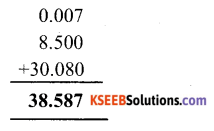b) 15 + 0.632 + 13.8:-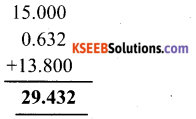c) 27.076 + 0.55 + 0.004:-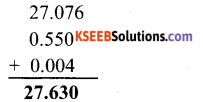d) 25.65 + 9.005 + 3.7:-e) 0.75 + 10.425 + 2:-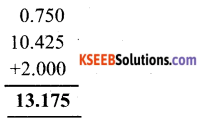f) 280.69 + 25.2 + 38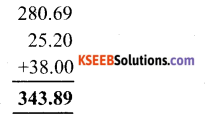Question 2.
Rashid spent Rs 35.75 for Maths book and Rs 32.60 for science book. Find the total amount spent by Rashid?
Solution: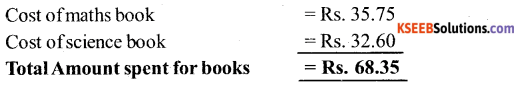The Amount spent by Rashid is Rs. 68.35

Question 3.
Radhika’s mother gave her Rs 10.50 and her father gave her Rs 15.80, Find the total amount given to Radhika by the parents.
Solution: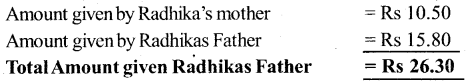Therefore, the amount given by her parents is Rs 26.30.Question 4.
Nasreen bought 3m 20 cm cloth for her shirt and 2 m 5 cm cloth for her trouser. Find the total length of cloth bought by her.
Solution:Hence, the total length of cloth bought by her is 5.25 mQuestion 5.
Naresh Walked 2 km 35m in the morning and 1 km 7 m in the evening. How much distance did he walk in all?
Solution:
Distance walked in the morning = 2 km 35m
Distance walked in the evening = 1 km 7m
1 km = 1000 m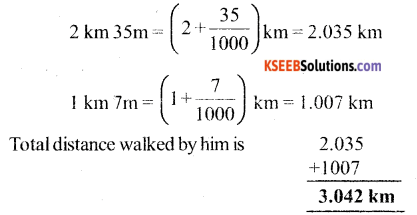Question 6.
Sunitha travelled 15 km 268 m by bus 7 km 7m by car and 500 m on foot in order to reach her school. How far is her school from her residence?
Solution:
W.K.T 1 km = 1000 mQuestion 7.
Ravi Purchases 5 kg 400 g rice, 2 kg 20g sugar and 10 kg 850 g flour. Find the total weight of his purchases?
Solution:error: Content is protected !!